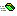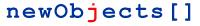Storages & Files TypeConvertor

The TypeConvertor object implements set of functions that extend the script languages. It is well-known that some script languages do not support some useful features - like detailed type conversion (JScript) or bit wise operations (VBScript). This object provides such methods. We will be glad to hear suggestions from you - we will add any other function often needed by the scripters but not available in all the scripting languages.Conv Syntax: variable = object.Conv(type, inVariable) Converts the inVariable to the type specified by the type parameter. It should be one variant type constants (see the AXPack1 constants)CBool Syntax: variable = object.CBool(inVariable) Converts the inVariable to Boolean (VT_BOOL)CByte Syntax: variable = object.CByte(inVariable) Converts the inVariable to Byte (VT_UI1)CCur Syntax: variable = object.CCur(inVariable) Converts the inVariable to Currency (VT_CY)CDate Syntax: variable = object.CDate(inVariable) Converts the inVariable to OLE date format (VT_DATE)CDbl Syntax: variable = object.CDbl(inVariable) Converts the inVariable to Double precision floating point value (VT_R8)CInt Syntax: variable = object.CInt(inVariable) Converts the inVariable to Integer (VT_I2). Warning to C++ programmers - this is equivalent to short. Automation tradition names the two byte signed integers just Integer.CLng Syntax: variable = object.CLng(inVariable) Converts the inVariable to Long Integer (VT_I4)CSng Syntax: variable = object.CSng(inVariable) Converts the inVariable to Single precision floating point value (VT_R4)CStr Syntax: variable = object.CStr(inVariable) Converts the inVariable to String (VT_BSTR)BitOr Syntax: variable = object.BitOr(x,y) Performs logical "Or" operation on bits of the passed parameters and returns the result. The parameters are first converted to Long Integers.BitAnd Syntax: variable = object.BitAnd(x,y) Performs logical "And" operation on bits of the passed parameters and returns the result. The parameters are first converted to Long Integers.BitXor Syntax: variable = object.BitXor(x,y) Performs logical "XOR" (eXclusive OR) operation on bits of the passed parameters and returns the result. The parameters are first converted to Long Integers.BitNeg Syntax: variable = object.BitNeg(x) Inverts the bits of  the passed parameter and returns the result. The parameter is first converted to Long Integer.BitLeft Syntax: variable = object.BitLeft(x,positions) Shifts the bits of the x left positions. The parameters are first converted to Long Integers.BitRight Syntax: variable = object.BitLeft(x,positions) Shifts the bits of the x right positions. The parameters are first converted to Long Integers.VariantType Syntax: variable = object.VariantType(v) Returns a number that specifies the type of the value v. You can test the returned value against the type constants.

...Copyright 2001-2006 newObjects [ ]